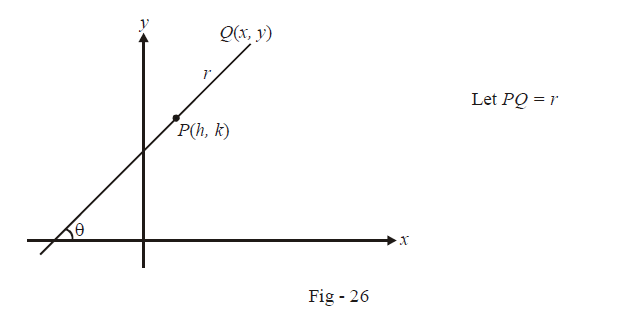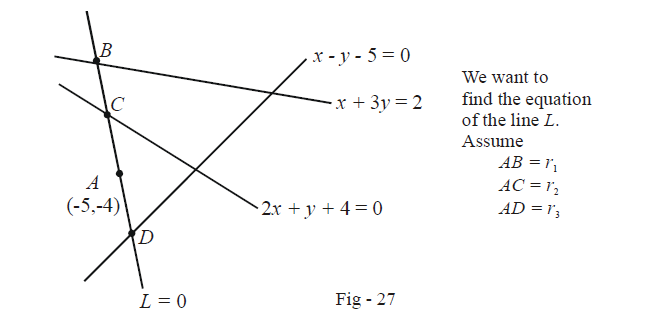# Polar or Distance Form of a Straight Line Equation

$$\textbf{Art 10 :} \qquad \boxed{{\text{Polar / Distance form of a line}}}$$

Sometimes, it is very convenient to write the equation of a straight line in polar / distance form. Suppose we know that the line passes through the fixed point $$P(h,\,k)$$ and is at an inclination of $$\theta :$$For any point $$Q(x,\,y)$$ at a distance r from along this line, we can write the simple relation

$\boxed{{\frac{{x - h}}{{\cos \theta }} = \frac{{y - k}}{{\sin \theta }} = r}}$

This is the required equation of the line. The point $$Q(x,\,y),$$ at a distance r from P, has the coordinates

$Q(x,\,y) \equiv (h + r\cos \theta ,\,k + r\sin \theta ).$

$\left\{ \begin{array}{l}{\text{Obviously, there will be another point, say }}Q'(x,y),{\text{ at a distance }}r{\text{ from }}P\\{\text{along this line but on the opposite side of }}Q{\text{; thus }}Q'(x,{\rm{ }}y){\text{ will have the }}\\{\text{coordinates }}Q'(x,\,y) \equiv (h - r\cos \theta ,\,\,\,k - r\sin \theta)\end{array} \right\}$

Example – 15

A line through $$A( - 5,\, - 4)$$ meets the lines $$x + 3y = 2,\,\,2x + y + 4 = 0$$ and $$x - y - 5 = 0$$ at the points B, C and D respectively. If

\begin{align}{\left( {\frac{{15}}{{AB}}} \right)^2} + {\left( {\frac{{10}}{{AC}}} \right)^2} = {\left( {\frac{6}{{AD}}} \right)^2},\end{align}

find the equation of the line.

Solution:The figure above roughly sketches the situation described in the equation. Let B, C and D be at distances $${r_1},\,{r_2}$$ and $${r_3}$$ from A along the line $$L = 0,$$ whose equation we wish to determine. Assume the inclination of L to be $$\theta .$$ Thus, B, C and D have the coordinates (respectively):

\begin{align}B \equiv ( - 5 + {r_1}\cos \theta ,\,\,\,\,\, - 4 + {r_1}\sin \theta )\\C \equiv ( - 5 + {r_2}\cos \theta ,\,\,\,\,\, - 4 + {r_2}\sin \theta )\\D \equiv ( - 5 + {r_3}\cos \theta ,\,\,\,\,\, - 4 + {r_3}\sin \theta )\end{align}

Since these three points(respectively) satisfy the three given equations, we have :

Point B\begin{align}( - 5 + {r_1}\cos \theta ) + 3( - 4 + {r_1}\sin \theta ) + 2 = 0 \quad \Rightarrow \qquad {r_1} = \frac{{15}}{{\cos \theta + 3\sin \theta }}\end{align}

Point C : \begin{align}2( - 5 + {r_2}\cos \theta ) + ( - 4 + {r_2}\sin \theta ) + 4 = 0 \quad \Rightarrow \qquad {r_2} = \frac{{10}}{{2\cos \theta + \sin \theta }}\end{align}

Point D : \begin{align}( - 5 + {r_3}\cos \theta ) - ( - 4 + {r_3}\sin \theta ) - 5 = 0 \quad \Rightarrow \qquad {r_3} = \frac{6}{{\cos \theta - \sin \theta }}\end{align}

It is given that

\begin{align}&{\left( {\frac{{15}}{{AB}}} \right)^2} + {\left( {\frac{{10}}{{AC}}} \right)^2} = {\left( {\frac{6}{{AD}}} \right)^2}\\ \text{i.e;}\qquad \qquad \qquad &{\left( {\frac{{15}}{{{r_1}}}} \right)^2} + {\left( {\frac{{10}}{{{r_2}}}} \right)^2} = {\left( {\frac{6}{{{r_3}}}} \right)^2}\\ \Rightarrow \qquad &{(\cos \theta + 3\sin \theta )^2} + {(2\cos \theta + \sin \theta )^2} = {(\cos \theta - \sin \theta )^2}\\ \Rightarrow \qquad &4{\cos ^2}\theta + 9{\sin ^2}\theta + 12\sin \theta \cos \theta = 0\\ \Rightarrow \qquad &{(2\cos \theta + 3\sin \theta )^2} = 0\\ \Rightarrow \qquad &\tan \theta = \frac{{ - 2}}{3}\\ \Rightarrow \qquad &m = \frac{{ - 2}}{3}\end{align}

Thus, we obtain the slope of as \begin{align}\frac{{ - 2}}{3}. \end{align} The equation of L can now be easily written :

\begin{align}&L:y - ( - 4) = \frac{{ - 2}}{3}(x - ( - 5))\\ \Rightarrow \qquad &L:2x + 3y + 22 = 0\end{align}

# TRY YOURSELF - I

Q1.  A variable straight line drawn through the intersection of the lines\begin{align}\frac{x}{a} + \frac{y}{b} = 1\;\;and\;\;\frac{x}{b} + \frac{y}{a} = 1\end{align} and meets the axes in A and B. Show that the locus of the mid-point of AB is $$2xy(a + b) = ab(x + y)$$

Q2. The line $$bx + ay = ab$$ cuts the axes in A and B. Another variable line cuts the axes in C and D such that $$OA + OB = OC + OD$$ where O is the origin. Prove that the locus of the point of intersection of the lines AD and BC is the line$$\;x + y = a + b$$

Q3.  A point P moves so that the square of its distance from (3, –2) is equal to its distance from the line $$5x - 12y = 13$$ Find the locus of P.

Q4.  A line intersects the x-axis in A(7, 0) and the y-axis in B(0, –5). A variable line perpendicular to AB intersects the x-axis in P and the y-axis in Q. If AQ and BP intersect in R, find the locus of R.

Q5. If the sum of the distances of a point from two perpendicular lines in a plane is 1, prove that its locus is a square.

Q6.  A vertex of an equilateral triangle is (2, 3) and the opposite side is $$\;x + y = 2$$.  Find the equations of the other sides.

Q7.  A ray of light along the line $$x - 2y - 3 = 0$$ is incident upon the mirror-line $$3x - 2y - 5 = 0$$ Find the equation of the reflected ray.

Q8. If the vertices of a triangle have integral coordinates, show that it cannot be equilateral.

Q9. Show using coordinate geometry that the angle bisectors of the sides of a triangle are concurrent.

Q10. The sides of a triangle are $$4x + 3y + 7 = 0\;,\;5x + 12y - 27 = 0\;\;and\;\;3x + 4y + 8 = 0$$ and By explicitly evaluating the medians in this triangle, show that they are concurrent.

Q11. A rod APB of constant length meets the axes in A and B. If AP = b and PB = a and the rod slides between the axes, show that the locus of P is$$\;{b^2}{x^2} + {a^2}{y^2} = {a^2}{b^2}$$

Q12. If p is the length of the perpendicular from the origin to the line whose intercepts on the axes are a and b, show that \begin{align}\frac{1}{{{p^2}}} = \frac{1}{{{a^2}}} + \frac{1}{{{b^2}}}\end{align}

Q13. The lines $$3x + 4y - 8 = 0\;and\;5x + 12y + 3 = 0$$ intersect in A. Find the equations of the lines passing through which intersect the given lines at B and C, such that $$AB = AC$$.

Q14. The equal sides AB and AC of an isosceles triangle ABC are produced to the points P and Q such that $$BP.CQ = A{B^2}$$ Prove that the line PQ always passes through a fixed point.

Q15. One side of a square is inclined to the x-axis at an angle and one of its extremities is at the origin; prove that the equations to its diagonals are

$\begin{array}{l} &y(\cos \alpha - \sin \alpha ) = x(\sin \alpha + \cos \alpha )\\\\ and\qquad &y(\sin \alpha + \cos \alpha ) + x(\cos \alpha - \sin \alpha ) = a \end{array}$

where a is the length of the side of the sqaure.